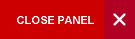## Introduction to Electronics

Semester 2

This course will adequately review the electronics content of CAPE Physics with extensions into slightly more advanced topics. Students will be introduced to basic concepts in analog and digital electronics, basic semiconductor theory, and analog communication systems (AM, FM, and PM). This course is required for all electronics engineering students and electronics majors and minors. The lab component for this course is offered as a separate teaching lab course (ELET1405).

Syllabus:
1. Introduction to Semiconductor Theory and the P-N Junction (13 hours):
• Review of the atomic structure and bonding, Conductor, insulator, and semiconductor; Semiconductor materials; Covalent bonded structures in semiconductor; Charge carriers and Energy levels; Energy level diagrams; Intrinsic and Extrinsic semiconductors; Doping; n-type and p-type semiconductors; Drift and Diffusion currents; Resistivity and conductivity; the Fermi Dirac Distribution function; The P-N Junction; P-N junction at Thermal Equilibrium; Junction capacitance; P-N junction diode; Characteristic curve of the p-n junction diode; Forward and reverse biasing; Diode circuits; Zener diodes; Diode data sheets; voltage doubler; Rectification: half wave and full wave; Light emitting diodes (LED); The Bipolar Junction Transistor (BJT); the Field Effect transistor; Biasing the transistor circuit; Transistor as a switch; Relay drivers; Logic gate design with transistors.
2. Introduction to Digital Electronics (13 hours):
• Analog and digital concepts; binary digits and logic levels; digital waveforms; logic gates and truth tables; physical realization of logic gates; Boolean algebra and logic simplification; DeMorgan’s theorem; Circuit minimization using Karnaugh maps; Terminologies used in logic designs: Fan in, Fan out, rise time, fall time, propagation delay; debounced switching; Combinational logic circuits:- Decoders, encoders, multiplexers, demultiplexers, parity generators, adders; Binary coded Decimal, Lathes, Flip Flops, application of Flip Flops; Storage circuits and devices-RAMS, Flash, volatile and non-volatile. ADC and DACs
3. Introduction to Analog Electronics and Communication Systems (13 hours):
• Introduction to alternating current (AC); Frequency dependent resistive (R), capacitive (C) and inductive (L) circuits; Resonance in RLC circuits; Determination of bandwidth and half-power points. The Operational Amplifier; Op amp characteristics; Feedback in op amp circuits; The inverting, summing and non-inverting amplifiers; The differentiator and the integrator; RC filters; First order active filters; Fundamentals of Communication Systems; Amplitude modulation (AM) and demodulation, Frequency modulation (FM) and demodulation, and Digital Communications basic, Basic building block of Transmitters and Receivers. Noise in communication systems.
Evaluation:

One 2-hour theory examination paper   60%

Two 1-hour in-course tests (2 x 20%)    40%

Learning Objectives:

The main objective of this course is to introduce students the basic theories of Electronics and its application to the design and analysis of basic analog, digital and semiconductor circuits.

After completing this course, students should be able tgn and evaluate operational amplifier circuits for applications to AC and DC amplification, first order filters, adders, integrators, and differentiators.

• Describe the structure and operation of the transistor.
• Demonstrate proficiency in determining bias voltages for transistor circuits.
• Describe the functional operation of simple AM and FM communication transmitters and receivers.
• Design and evaluate logic circuits for applications to various combinational logic circuit design, flip flops, decoders, encoders, multiplexers, demultiplexers, parity and adders.
• Describe the differences between conductor, insulator and semiconductor in terms of their energy levels
• Describe the formation of the p-n junction via energy level diagrams and physical structure
• Design and evaluate p-n junction diode circuits for applications to rectifiers, voltage doublers, and voltage regulators.
CAPE/A-Level Physics or P04A/PHYS0410 and P04B/PHYS0420 or CSEC Physics with CAPE/A-Level Maths or M08B/MATH0100 and M08C/MATH0110 or ELNG1101

Required Textbook:

Boylestad and Nashelsky; Electronic Devices and Circuit Theory; Pearson Publication, 2009.

Thomas Floyd; Digital Electronics; 9th Ed, Pearson Publication, 2008

S. Pooranchandra, B. Sasikala, and Afzal Khan;  “Introduction to Electrical, Electronics, and Communication Engineering” Laxmi Publications;  December, 2005

Reference:

Introduction to Electronics” – by Earl Gates; Delmar Cengage Learning; 5th Edition; May 24, 2006

Internet Sources:

1. Sections of text online: http://books.google.com.jm/books?id=f5PirPttxpAC&printsec=frontcover&dq=Introduction+to+Electrical,+Electronics,+and+Communication+Engineering#PPP1,M1
2. Online tutorials: http://michaelgellis.tripod.com/tutorial.html
3. Online tutorials: http://www.electronics-tutorials.com/
Course Code:
ELET1400
Credits:
3 Credits
Level:
Level 1
Top of Page